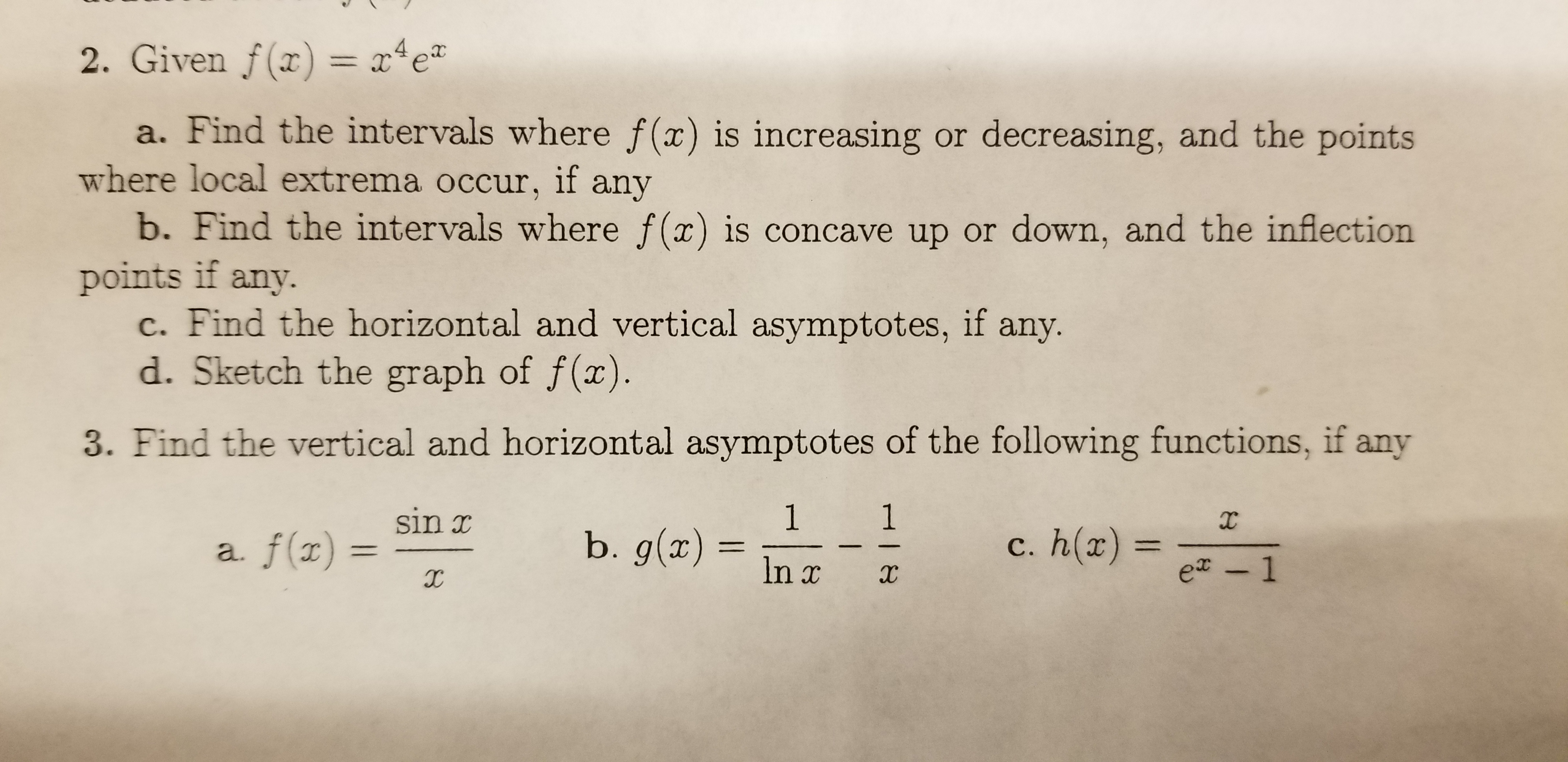# 2. Given f(x) = x4ea. Find the intervals where f(x) is increasing or decreasing, and the pointswhere local extrema occur, if anyb. Find the intervals where f(x) is concave up or down, and the inflectionpoints if any.c. Find the horizontal and vertical asymptotes, ifd. Sketch the graph of f(x).any.3. Find the vertical and horizontal asymptotes of the following functions, if any1b. g(x)1sin xXc. h(x) =a. f(x11e - 1In xHI8

Question
15 views

Question 2 Part A, B, C, & D. Thank you!help_outlineImage Transcriptionclose2. Given f(x) = x4e a. Find the intervals where f(x) is increasing or decreasing, and the points where local extrema occur, if any b. Find the intervals where f(x) is concave up or down, and the inflection points if any. c. Find the horizontal and vertical asymptotes, if d. Sketch the graph of f(x). any. 3. Find the vertical and horizontal asymptotes of the following functions, if any 1 b. g(x) 1 sin x X c. h(x) = a. f(x 11 e - 1 In x HI8 fullscreen
check_circle

Step 1

Note:

Since you have posted a question with multiple sub-parts, we will solve first subpart for you. To get remaining sub-part solved please repost the question separately.

Step 2

Differentiate the given function

Step 3

Obtain the critic...

### Want to see the full answer?

See Solution

#### Want to see this answer and more?

Solutions are written by subject experts who are available 24/7. Questions are typically answered within 1 hour.*

See Solution
*Response times may vary by subject and question.
Tagged in

### Derivative